# Unknown numbers

The sum of two consecutive natural numbers and their triple is 92. Find these numbers.

a =  11
b =  12

### Step-by-step explanation:

b = a+1
a+b + 3(a+b) = 92

b = a+1
a+b + 3•(a+b) = 92

a-b = -1
4a+4b = 92

a = 11
b = 12

Our linear equations calculator calculates it.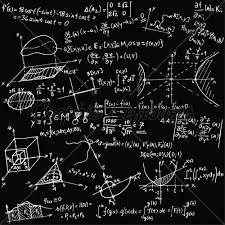Did you find an error or inaccuracy? Feel free to write us. Thank you!Tips to related online calculators
Do you have a linear equation or system of equations and looking for its solution? Or do you have a quadratic equation?

## Related math problems and questions:

• Have solutionThe sum of four consecutive even numbers is 92. Determine these numbers.
• Find twoFind two consecutive natural numbers whose product is 1 larger than their sum. Searched numbers expressed by a fraction whose numerator is the difference between these numbers and the denominator is their sum.
• Odd numbersThe sum of four consecutive odd numbers is 1048. Find those numbers ...
• Two numbers 7The sum of two consecutive even numbers is 30. Find the numbers.
• Sum of sevenThe sum of seven consecutive odd natural numbers is 119. Determine the smallest of them.
• Ratio of two unknown numbersTwo numbers are given. Their sum is 30. We calculate one-sixth of a larger number and add to both numbers. So we get new numbers whose ratio is 5:7. Which two numbers were given?
• Unknown numberIf I reduced the sum of the numbers 70 and the unknown number three times, I would get 100. what is the unknown number?
• Lcm = 22 + gcdThe least common multiple of two numbers is 22 more than their greatest common divisor. Find these numbers.
• Two numbersFind two numbers whose difference and ratio is 2.
• Unknown number 716% of the unknown number is by 21 less than unknown number itself. Determine the natural unknown number.
• Ratio three numbers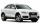Three numbers SUV are in the ratio 1:2:3. Their sum is 24. Find this numbers and write their add and sum.
• Even / odd numbers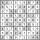a / Using variable n write two consecutive odd numbers. b / the sum of three consecutive odd numbers is 333. What are this numbers?
• Unknown numberFind an unknown number of which 1/5 is 40 greater than one-tenth of that number.
• Summands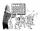Find two summands of the number 42, so that its product is minimized.
• Real estate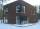The residential house has three entrances numbered by odd numbers arithmetic progression. The sum of the two numbers on the corner entrances is 50. Calculate the highest of these three numbers.
• Sum of three numbersThe sum of three numbers from which second number is 20% smaller than the first number and the third number is 25% smaller than the second number is 96. Determine this numbers.
• Sum-log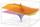The sum of two numbers is 32, the sum of their logarithms (base 10) is 2.2. Determine these numbers.